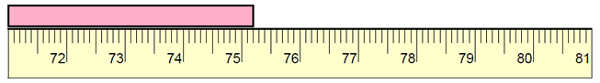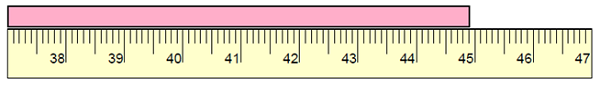# Measuring Length to the Nearest Millimeter

#### Complete Python Prime Pack

9 Courses     2 eBooks

#### Artificial Intelligence & Machine Learning Prime Pack

6 Courses     1 eBooks

#### Java Prime Pack

9 Courses     2 eBooks

Small units of lengths are called millimeters. The thickness of a credit card or the thickness of say 10 white sheets of paper are of the order of a millimeter in length.

In this lesson, we solve problems where the length of objects is measured to the nearest millimeter. This means that the measurement is accurate up to a millimeter. Usually insects like fleas, red ants have lengths of the order of millimeters.

Find the length of the following object to the nearest millimeter.### Solution

Step 1:

The reading is closer to 729 mm than to 728 mm.

Step 2:

So, the length of the given object to the nearest centimeter is 729 mm.

Find the length of the following object to the nearest millimeter.### Solution

Step 1:

The reading is closer to 752 mm than to 751 mm.

Step 2:

So, the length of the given object to the nearest centimeter is 752 mm.

Find the length of the following object to the nearest millimeter.### Solution

Step 1:

The reading is closer to 449 mm than to 448 mm.

Step 2:

So, the length of the given object to the nearest centimeter is 449 mm.